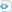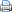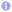C++

# Revisiting the named parameter idiom in C++14

Some programming languages have functions with named parameters. Named parameters let the programmer pass the parameters to a function in any order and they are distinguished by a name. So the programmer can explicitly pass all the needed parameters and default values without worrying about the order used in the function declaration. In C++, however, functions have positional arguments.  This means, for functions with many parameters, the programmer has to remember the types and the order in which to pass them. Furthermore, default values can only be given to the last parameters, so it is not possible to specify one of the later parameters and use the default value for former ones.

Named parameters might become a feature of future C++ versions but can not jet be used directly in C++. Thus, C++ programmers have devised various techniques to emulate named parameters in C++, e.g., by using method chaining. C++14 offers some new features which allow implementations of named parameters with a very neat syntax, as I will demonstrate in the following. The presented implementation ensures that some possible errors, which might occur in the context of named parameters are detected at compile time.

• Each named parameter can be given only once per function call.
• Named parameters without a default value must be given.
• Only allowed named parameters can be passed to a function.

Let us have a look at a specific example how this implementation can be used. In this implementation, parameter names and values are represented by structures with a single data member value. In the following example these are name, buffersize and writemode. These structures are passed as anonymous objects, which are constructed via aggregate initialization, to a variadic template function, which forwards these function arguments (or the default values) in the correct order to the actual implementation by a standard function with a fixed number of positional arguments.

 Source code#include <iostream>
#include <string>
#include "named_parameter.hpp"

// some structures representing parameter names and values
struct name {
const std::string &value;
};

struct buffersize {
const int &value;
};

struct writemode {
const bool value;
};

constexpr writemode writable{true};

struct invalid {
const int &value;
};

// a function with many parameters
void open_file_impl(const std::string &name, int buffersize, bool writable) {
std::cout << "name       : " << name << std::endl;
std::cout << "buffersize : " << buffersize << std::endl;
std::cout << "writable   : " << writable << std::endl;
std::cout << std::endl;
}

// template function taking named parameters which
// are passed to actual function implementation
template<typename... Ts>
void open_file(const Ts &...args) {
static_assert(named_para::is_valid<named_para::parameter_set<name, buffersize, writemode>, Ts...>(),
"invalid named parameter argument");
auto t=std::make_tuple(args...);
open_file_impl(named_para::get<name>(t),
named_para::get<buffersize>(t, 1024),
named_para::get<writemode>(t, false));
}

int main() {
open_file(name{"file.dat"});
open_file(buffersize{8192}, writable, name{"file_name.dat"});
// next line will not compile because of missing mandatory parameter
// open_file(buffersize{1234});
// next line will not compile because of invalid parameter
// open_file(buffersize{1234}, name{"another_file_name.dat"}, invalid{1});
// next line will not compile because of repeated parameter
// open_file(buffersize{1234}, name{"another_file_name.dat"}, name{"foo"});
}

The implementation of the named parameters is given in the following header file. The whole magic of this implementation relies on the template class std::tuple, which allows in C++14 to access a specific element via its type by the function std::get, and variadic templates. In the application code as shown above, function arguments to the variadic template function are put into a std::tuple and the function named_para::get returns a desired function argument from the tuple or a possible default value if not present. In this way, named function arguments can be put into the right order to pass them to a function with positional arguments. In order to decide if the default argument has to be employed, it has to be checked if a certain type, which represents a parameter name, is present in the set of given arguments. This is accomplished by the template class has_type, which has either std::true or std::false as base class, depending on if the parameter name is present in the function call or not. Finally, the helper class is_valid can be used to check if a set of given parameter names contains only valid types (parameter names).

 Source code#ifndef NAMED_PARAMETER_HPP
#define NAMED_PARAMETER_HPP

#include <tuple>
#include <type_traits>

namespace named_para {

// compile time check if type T is in tuple of type Tuple
template<typename T, typename Tuple>
struct has_type;

// empty tuple cannot contain T
template<typename T>
struct has_type<T, std::tuple<>> : std::false_type {
};

// 1st type in tuple is not T, T may be in other elements
template<typename T, typename U, typename... Ts>
struct has_type<T, std::tuple<U, Ts...>> : has_type<T, std::tuple<Ts...>> {
};

// 1st type in tuple is T, thus tuple contains an element of type T
template<typename T, typename... Ts>
struct has_type<T, std::tuple<T, Ts...>> : std::true_type {
};

// compile time check if type pack Ts contains only valid types given in tuple
template<typename Tuple, typename... Ts>
class is_valid;

// check if T is in Tuple if type pack has only a single type T
template<typename Tuple, typename T>
struct is_valid<Tuple, T> : has_type<T, Tuple> {
};

// recursive definition of is_valid
// type pack is valid if 1st element is valid and remaining types are valid
template<typename Tuple, typename T, typename... Ts>
struct is_valid<Tuple, T, Ts...> : std::integral_constant<bool, is_valid<Tuple, T>() and is_valid<Tuple, Ts...>()> {
};

template<typename T, typename Tup, typename Enable=void>
class get_impl {
public:
// type T not present in tupe, return default value
static auto get(const Tup &tup, const decltype(T::value) &def) -> decltype(T::value) {
return def;
}
};

template<typename T, typename Tup>
class get_impl<T, Tup, typename std::enable_if<has_type<T, Tup>::value>::type> {
public:
// type T present in tuple, return T::value from tuple, ignore 2nd parameter
static auto get(const Tup &tup, const decltype(T::value) &) -> decltype(T::value) {
return std::get<T>(tup).value;
}
// type T present in tuple, return T::value from tuple
static auto get(const Tup &tup) -> decltype(T::value) {
return std::get<T>(tup).value;
}
};

// get T::value if element of type T present in tuple or default parameter otherwise
template<typename T, typename Tup>
auto get(const Tup &tup, const decltype(T::value) &def) -> decltype(get_impl<T, Tup>::get(tup, def)) {
return get_impl<T, Tup>::get(tup, def);
};

// get T::value from tuple
template<typename T, typename Tup>
auto get(const Tup &tup) -> decltype(get_impl<T, Tup>::get(tup)) {
return get_impl<T, Tup>::get(tup);
};

template<typename ...Ts> using parameter_set = std::tuple<Ts...>;
}

#endif